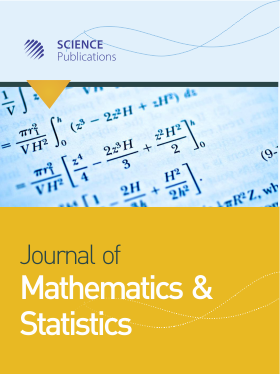Frequency: Continuous
ISSN: 1549-3644 (Print)
ISSN: 1558-6359 (Online)
Research Article Open Access

# On Gröbner Bases and Their Uses in Solving System of Polynomial Equations and Graph Coloring

Haridas Kumar Das1 and Nasim Reza1
• 1 University of Dhaka, Bangladesh

## Abstract

This paper is based on the analytic and computational solution procedures of Gröbner basis and its applications. We show the behavior of the ideals generated by polynomials from a polynomial ring. We also present the idea of a zero dimensional ideal and use of this ideal to solve system of polynomial equations. We then introduce an algorithmic procedure for solving a system of polynomial equations (linear and nonlinear) with a finite number of solutions extending the idea of Gröbner basis. Finally we explore the idea of Gröbner basis for coloring the vertices of a given graph. We illustrate the stated results through a number of examples. Moreover, as for auxilary and making comparison with the analytic results, we use Mathematica 9.0.1 to develop some computer algebra.

Journal of Mathematics and Statistics
Volume 14 No. 1, 2018, 175-182

DOI:

Submitted On: 30 March 2018 Published On: 27 June 2018

How to Cite: Das, H. K. & Reza, N. (2018). On Gröbner Bases and Their Uses in Solving System of Polynomial Equations and Graph Coloring. Journal of Mathematics and Statistics, 14(1), 175-182. https://doi.org/10.3844/jmssp.2018.175.182

• 1,617 Views